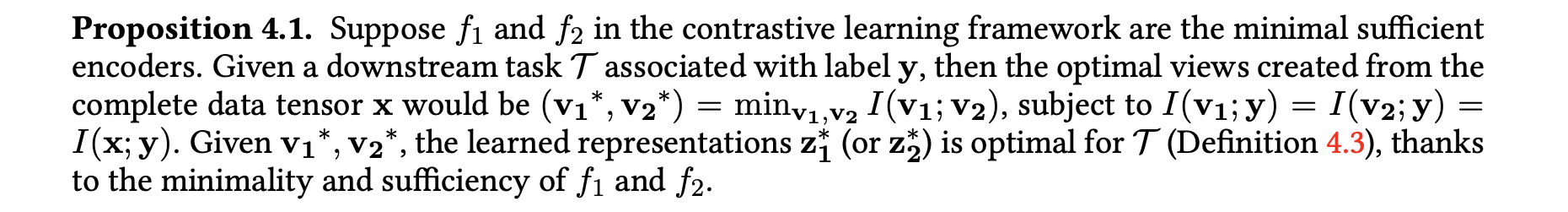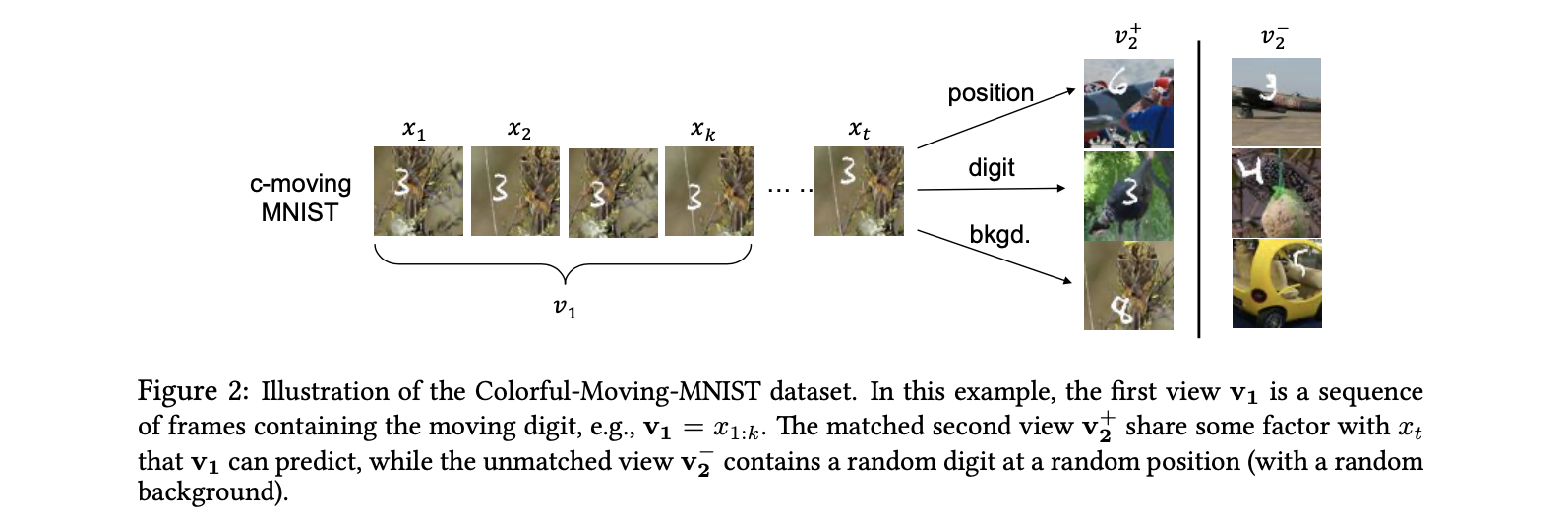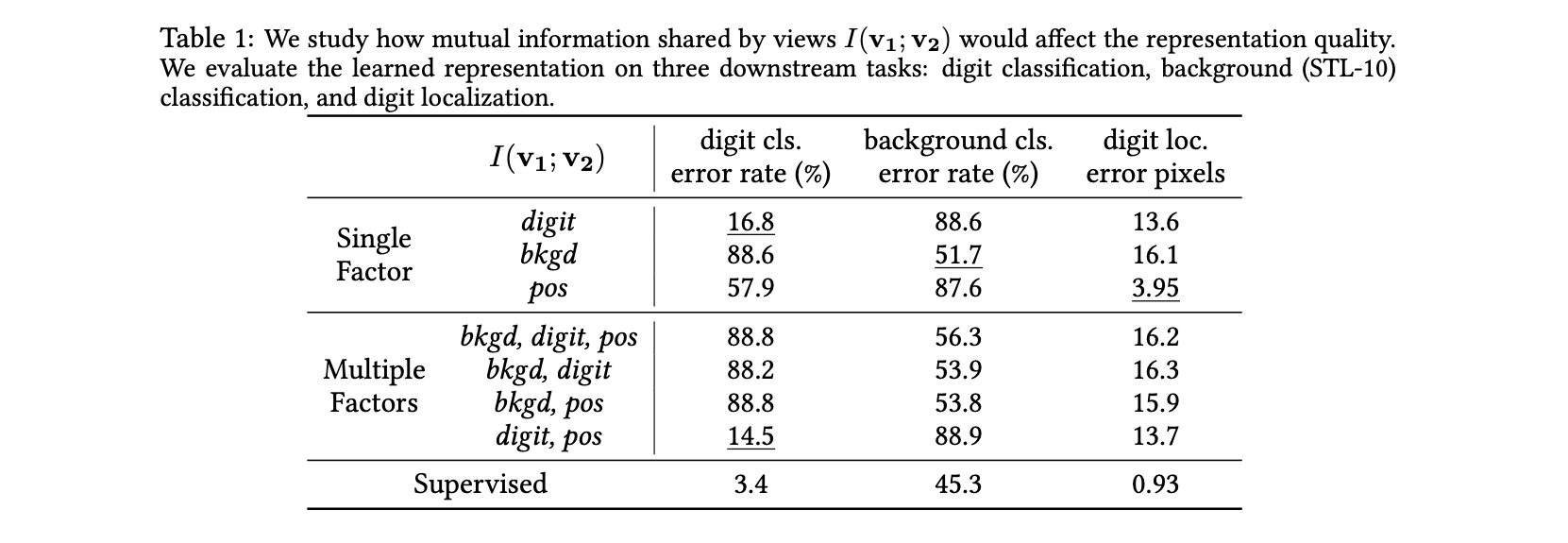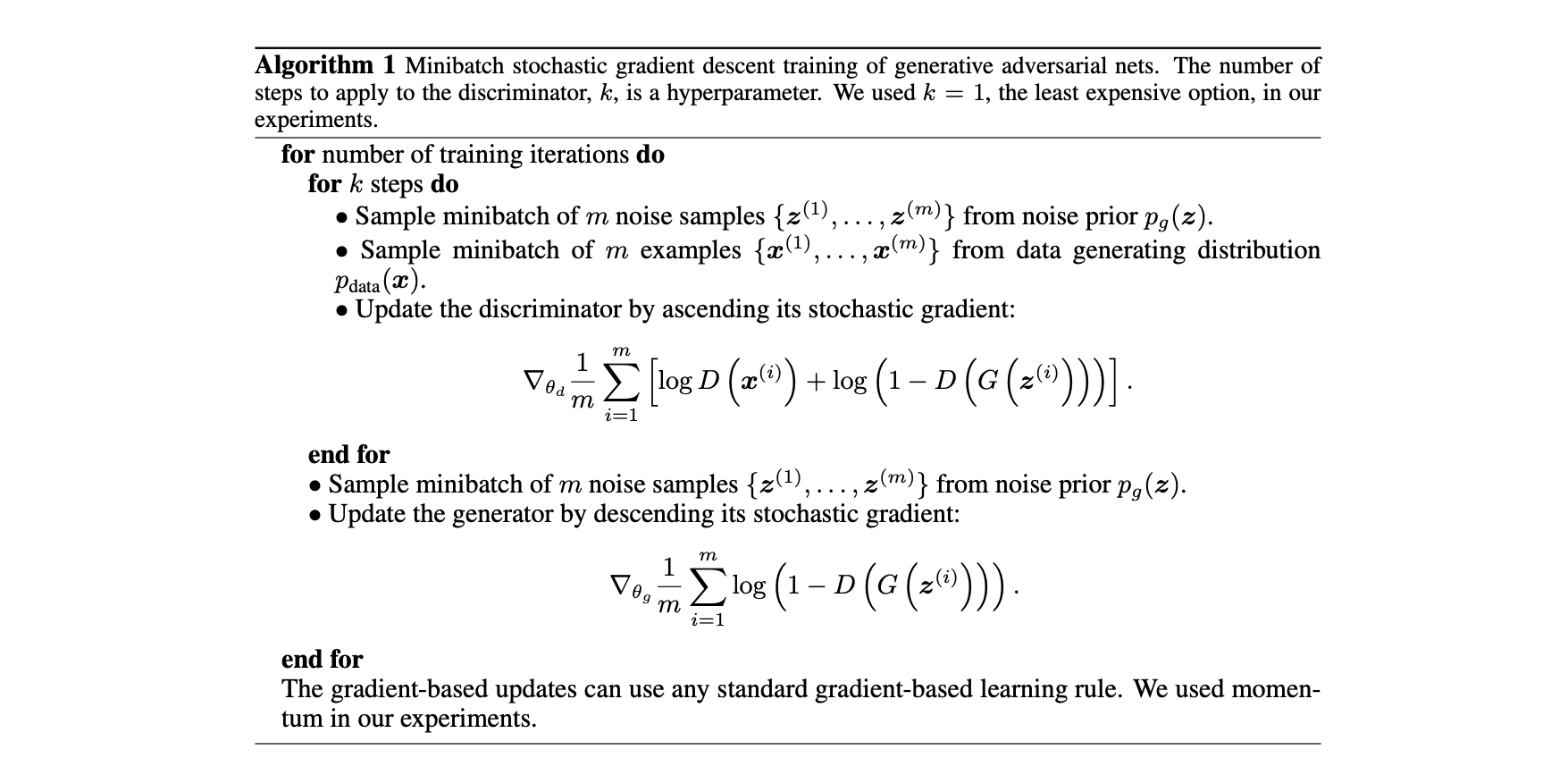N1H111SM's Miniverse

# What Makes for Good Views for Contrastive Learning

2020/05/26 ShareMaterials

# Motivation

Despite the success of the Contrastive Multiview Coding (CMC), the influence of different view choices has been less studied. In this paper, we use empirical analysis to better understand the importance of view selection, and argue that we should reduce the mutual information (MI) between views while keeping task-relevant information intact. To verify this hypothesis, we devise unsupervised and semi-supervised frameworks that learn effective views by aiming to reduce their MI.

## Structure of Introduction

• CMC relies on the fundamental assumption that important information is share across views, which means it’s view-invariant.
• Then which viewing conditions should it be invariant to?
• We therefore seek representations with enough invariance to be robust to inconsequential variations but not so much as to discard information required by downstream tasks.
• We investigate this question in two ways.
• Optimal choice of views depends critically on the downstream task.
• For many common ways of generating views, there is a sweet spot in terms of downstream performance where the mutual information (MI) between views is neither too high nor too low.
• InfoMin principle: A good set of views are those that share the minimal information necessary to perform well at the downstream task.

# Methods

Definition 4.1. (Sufficient Encoder) The encoder $f_1$ of $v_1$ is sufficient in the contrastive learning framework if and only if $I(v_1; v_2) = I(f_1(v_1); v_2)$.

Definition 4.2. (Minimal Sufficient Encoder) A sufficient encoder $f_1$ of $v_1$ is minimal if and only if $I(f_1(v_1);v_1) \leq I(f(v_1);v_1) \forall f$, that are sufficient. Among those encoders which are sufficient, the minimal ones only extract relevant information of the contrastive task and will throw away other information.

Definition 4.3. (Optimal Representation of a Task) For a task $\mathcal T$ whose goal is to predict a semantic label $y$ from the input data $x$, the optimal representation $z^\star$ encoded from $x$ is the minimal sufficient statistic with respect to $y$. 以上说明了$z^\star$保留了所有用于和task $\mathcal T$相关的信息，因此被称作optimal的。

## InfoMin Principle## Unsupervised InfoMin